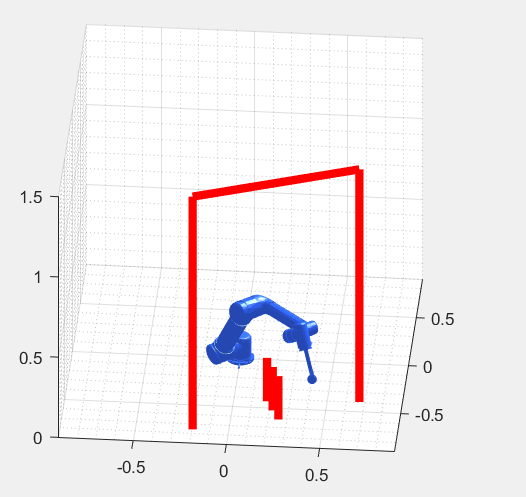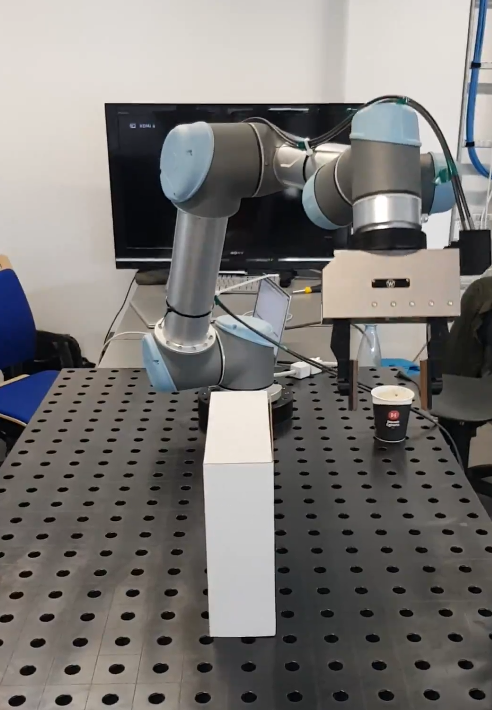# Final Project - SDU Robotics Summer Course

Implementation of two motion planning algorithms:

• Rapidly-exploring Random Tree (RRT)
• Potential Field

The project report can be found hereBoth algorithms find collision-free paths for the UR5 robotic arm (Universal Robots site) to perform pick-and-place tasks.

Scripts MPExtendRRT.m and PotentialField.m display a simulation of the UR5 robot following the path found and the obstacles defined.

A *.script output file is generated with the commands for the real UR5 robot. Example:

``````...
movej([-0.83856, -1.96147, -1.06408, -1.06838, 1.58115, -1.01197], a=1, v=1, t=0, r=0)
movej([-0.834858, -2.04677, -1.06577, -1.07883, 1.61465, -0.997554], a=1, v=1, t=0, r=0)
movej([-0.860245, -2.12685, -1.0378, -1.09263, 1.64701, -0.967192], a=1, v=1, t=0, r=0)
movej([-0.83583, -2.1846, -1.08443, -1.11736, 1.62908, -1.02161], a=1, v=1, t=0, r=0)
...
``````Video of the real UR5 robot following the generated path using the RRT algorithm

### Usage

Set up a a MEX compiler:

``````mex -setup C++
``````

Initialize toolbox parameters:

``````run_me
``````

``````load demo_vars.mat
``````

Run motion planning algorithm:

``````MPExtendRRT(initial_configuration, goal_configuration, obstacles)
PotentialField(initial_configuration, goal_configuration, obstacles)
``````

initial_configuration is the initial configuration of UR5 (dimension: 1*6, unit: radian)

goal_configuration is the goal configuration of UR5 (dimension: 1*6, unit: radian)

obstacles represents all the capsule obstacles in the workspace (dimension: n*7)
format of each row of obstacles: [P_ini, P_end, r] where P_ini is the x-, y- and z- coordinates of the initial point of the interal line segment of the capsule (diemsion: 1*3 unit: meter), P_end is the x-, y- and z- coordinates of the end point of the interal line segment of the capsule (diemsion: 1*3 unit: meter), r is the radius of the capsule (scalar, unit: meter)